library(prodest)
library(tidyverse)

Panel Simulation

Source for the below Econometrics by Simulation:

It is common for researchers to be concerned about unobserved effects being correlated with observed explanatory variables.

For instance, if we were curious about the effect of meditation on emotional stability we may be concerned that there might be some unobserved factor such as personal genetics that might predict both likelihood to meditate and emotional stability.

In order to remove this potentially biasing effect we could think about taking measurements over multiple periods for the same individual inquiring about frequency of meditation and emotional stability.

If we observe that within the same individual, removing the time constant effects which (presumably) genetics is a component of that there is still a relationship between meditation and emotional stability, then we may feel on firmer ground as to our hypothesis that mediation may lead to more emotional stability.

In order to accomplish the goal of estimating this relationship we may experiment with a “fixed effects” model defined as:

$y_{it}=x_{it}\beta + a_i+u_{it}$

In this typical linear model with panel data, there is no problem including an arbitrary number of dummy variables. Let’s see this in action.

set.seed(1234)
nperson <- 5 # Number of persons
nobs <- 300      # Number of observations per person

In order for unobserved person effects to be a problem they must be correlated with the explanatory variable. Let’s say: x = x.base + fe

fe.sd <- 1 # Spefify the standard deviation of the fixed effed
x.sd  <- 1 # Specify the base standard deviation of x

beta <- 2

# First generate our data using the time constant effects
constantdata <- data.frame(id=1:nperson, fe=rnorm(nperson))

# We expand our data by nobs
fulldata <- constantdata[rep(1:nperson, each=nobs),]

Add a time index, first define a group apply function that applies by group index.

gapply <- function(x, group, fun) {
returner <- numeric(length(group))
for (i in unique(group))
returner[i==group] <- get("fun")(x[i==group])
returner
}

Using the generalized apply function coded above:

fulldata$t <- gapply(rep(1,length(fulldata$id)),
group=fulldata$id, fun=cumsum) # Or a more simplified function indexer <- function(group) { returner <- numeric(length(group)) for (i in unique(group)) returner[i==group] <- 1:sum(i==group) returner } # Is a special case of gapply fulldata$t <- indexer(fulldata$id) # Now we are ready to caculate the time variant xs fulldata$x <- fulldata$fe + rnorm(nobs*nperson) # And our unobservable error fulldata$u <- rnorm(nobs*nperson)
true_beta <- 2
fe_coefficient <- 30
# Finally we are ready to simulate our y variables
fulldata$y <- true_beta*fulldata$x + fe_coefficient*fulldata$fe + fulldata$u

Plotting

fulldata %>%
select(id,t,x,y) %>%
mutate(id = factor(id)) %>%
pivot_longer(cols = c(-id,-t),names_to="variable",values_to ="value") %>%

ggplot(aes(x=t,y=value,color=id)) +
geom_line() +
facet_wrap(~variable)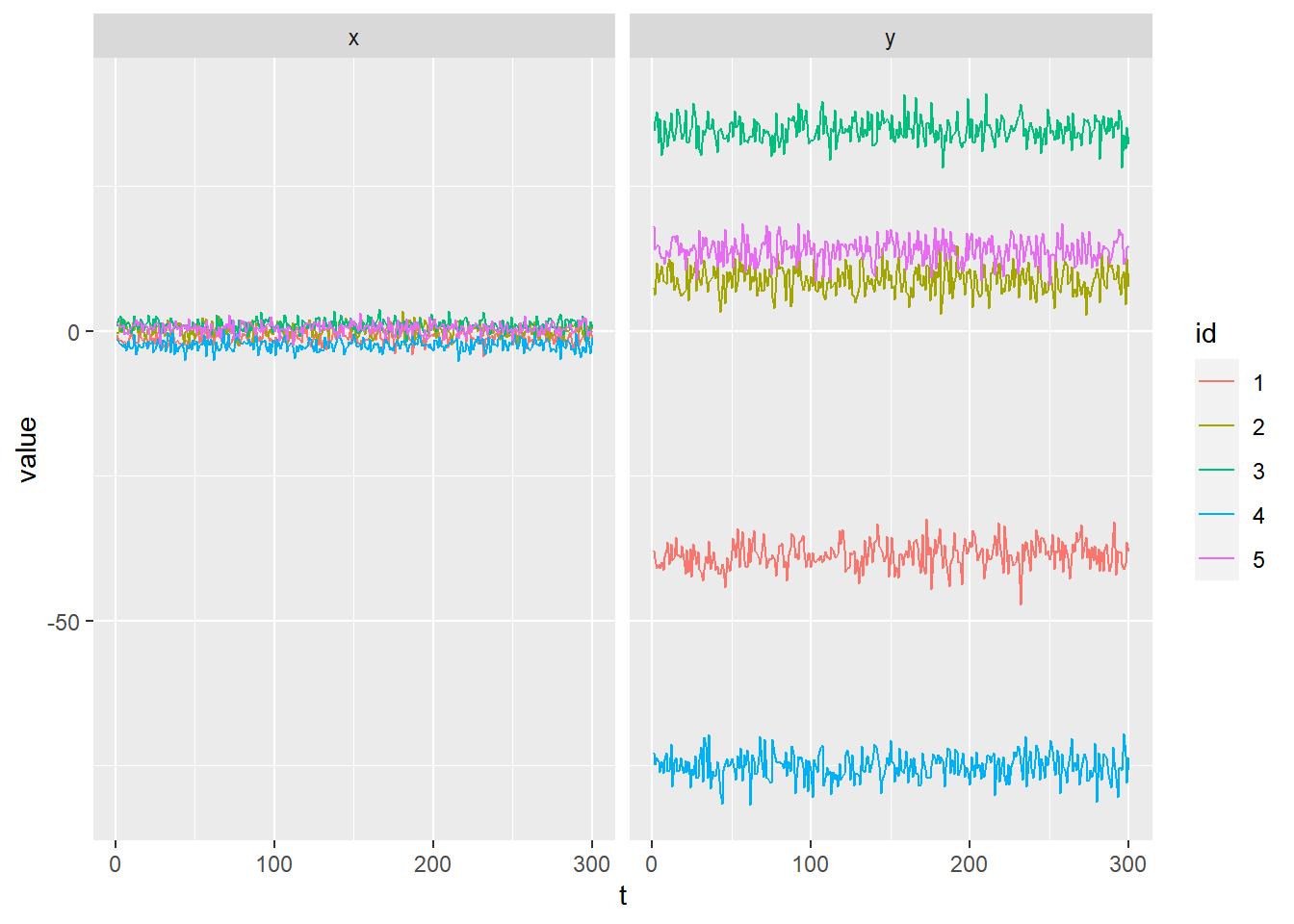Model Results

# First lets see how our standard linear model performs:
summary(lm(y~x, data=fulldata))

Call:
lm(formula = y ~ x, data = fulldata)

Residuals:
Min      1Q  Median      3Q     Max
-68.334 -15.600   0.409  15.855  72.441

Coefficients:
Estimate Std. Error t value Pr(>|t|)
(Intercept)  -3.8672     0.6105  -6.334 3.14e-10 ***
x            20.6060     0.3753  54.905  < 2e-16 ***
---
Signif. codes:  0 '***' 0.001 '**' 0.01 '*' 0.05 '.' 0.1 ' ' 1

Residual standard error: 23.06 on 1498 degrees of freedom
Multiple R-squared:  0.668, Adjusted R-squared:  0.6678
F-statistic:  3015 on 1 and 1498 DF,  p-value: < 2.2e-16
# Adding a dummy variable removes the bias
summary(lm(y~x+factor(id), data=fulldata))

Call:
lm(formula = y ~ x + factor(id), data = fulldata)

Residuals:
Min       1Q   Median       3Q      Max
-3.12130 -0.70333  0.00404  0.68725  3.11956

Coefficients:
Estimate Std. Error t value Pr(>|t|)
(Intercept) -36.21893    0.06685 -541.76   <2e-16 ***
x             2.03188    0.02690   75.53   <2e-16 ***
factor(id)2  44.59704    0.09130  488.45   <2e-16 ***
factor(id)3  68.76439    0.10250  670.90   <2e-16 ***
factor(id)4 -34.06748    0.08894 -383.06   <2e-16 ***
factor(id)5  49.11367    0.09469  518.70   <2e-16 ***
---
Signif. codes:  0 '***' 0.001 '**' 0.01 '*' 0.05 '.' 0.1 ' ' 1

Residual standard error: 1.016 on 1494 degrees of freedom
Multiple R-squared:  0.9994,    Adjusted R-squared:  0.9994
F-statistic: 4.642e+05 on 5 and 1494 DF,  p-value: < 2.2e-16

The same result can be taken by removing the mean from both the explanatory variables and the dependent variables. Why is that?

Think of the problem as: $y_{it}=x_{it}\beta + a_i+u_{it}$ So $y_{it}-mean_t(y_i)=(x_{it}-mean_t(x_{i}))\beta + a_i-mean_t(a_i)+u_{it}-mean(u_i)$

Because the unobservable effect is constant over time it drops out. And so long as their was a term controlling for the average unobservable effect (the dummy variables) then the average per person unobserved error must by definition be equal to zero.

thus: $y_{it}-mean_t(y_i)=(x_{it}-mean_t(x_{i}))\beta + u_{it}$

fulldata$ydemean <- fulldata$y-ave(fulldata$y, group=fulldata$id)
fulldata$xdemean <- fulldata$x-ave(fulldata$x, group=fulldata$id)

summary(lm(ydemean~xdemean-1, data=fulldata))

Call:
lm(formula = ydemean ~ xdemean - 1, data = fulldata)

Residuals:
Min       1Q   Median       3Q      Max
-3.12130 -0.70333  0.00404  0.68725  3.11956

Coefficients:
Estimate Std. Error t value Pr(>|t|)
xdemean  2.03188    0.02686   75.66   <2e-16 ***
---
Signif. codes:  0 '***' 0.001 '**' 0.01 '*' 0.05 '.' 0.1 ' ' 1

Residual standard error: 1.015 on 1499 degrees of freedom
Multiple R-squared:  0.7925,    Adjusted R-squared:  0.7923
F-statistic:  5724 on 1 and 1499 DF,  p-value: < 2.2e-16

We can also accomplish this by adding the Chamberlain device to the that regression is the total or mean of the explanatory variables at the level of each individual.

fulldata$xmean <- ave(fulldata$x, group=fulldata$id) fulldata$xsum <- gapply(fulldata$x, group=fulldata$id, fun=sum)

This is a little trickier to figure out how it accomplishes the task of differencing out the unobserved effect. This is how I think of it. The unobserved individual effect must be correlated with the explanatory variable in aggregate to be a problem.However, that correlation can only be on the individual level since by definition the “fixed effect” is constant on the individual level. Thus by creating a new variable which is the average or total for each individual, we are allocating to that variable any variation which correlates with the explanatory variable.

summary(lm(y~x+xmean, data=fulldata))

Call:
lm(formula = y ~ x + xmean, data = fulldata)

Residuals:
Min      1Q  Median      3Q     Max
-5.1726 -1.2352  0.2003  1.2902  4.6120

Coefficients:
Estimate Std. Error t value Pr(>|t|)
(Intercept)  0.18886    0.04688   4.029 5.89e-05 ***
x            2.03188    0.04619  43.994  < 2e-16 ***
xmean       29.86872    0.05857 509.989  < 2e-16 ***
---
Signif. codes:  0 '***' 0.001 '**' 0.01 '*' 0.05 '.' 0.1 ' ' 1

Residual standard error: 1.745 on 1497 degrees of freedom
Multiple R-squared:  0.9981,    Adjusted R-squared:  0.9981
F-statistic: 3.933e+05 on 2 and 1497 DF,  p-value: < 2.2e-16
summary(lm(y~x+xsum, data=fulldata))

Call:
lm(formula = y ~ x + xsum, data = fulldata)

Residuals:
Min      1Q  Median      3Q     Max
-5.1726 -1.2352  0.2003  1.2902  4.6120

Coefficients:
Estimate Std. Error t value Pr(>|t|)
(Intercept) 0.1888619  0.0468788   4.029 5.89e-05 ***
x           2.0318811  0.0461850  43.994  < 2e-16 ***
xsum        0.0995624  0.0001952 509.989  < 2e-16 ***
---
Signif. codes:  0 '***' 0.001 '**' 0.01 '*' 0.05 '.' 0.1 ' ' 1

Residual standard error: 1.745 on 1497 degrees of freedom
Multiple R-squared:  0.9981,    Adjusted R-squared:  0.9981
F-statistic: 3.933e+05 on 2 and 1497 DF,  p-value: < 2.2e-16

Outliers

A. Specific Outlier in x

A <- fulldata
A %>%
mutate(x = ifelse(id == 4 & t== 270,x+20,x)) -> A

A %>%
select(id,t,x,y) %>%
mutate(id = factor(id)) %>%
pivot_longer(cols = c(-id,-t),names_to="variable",values_to ="value") %>%

ggplot(aes(x=t,y=value,color=id)) +
geom_line() +
facet_wrap(~variable)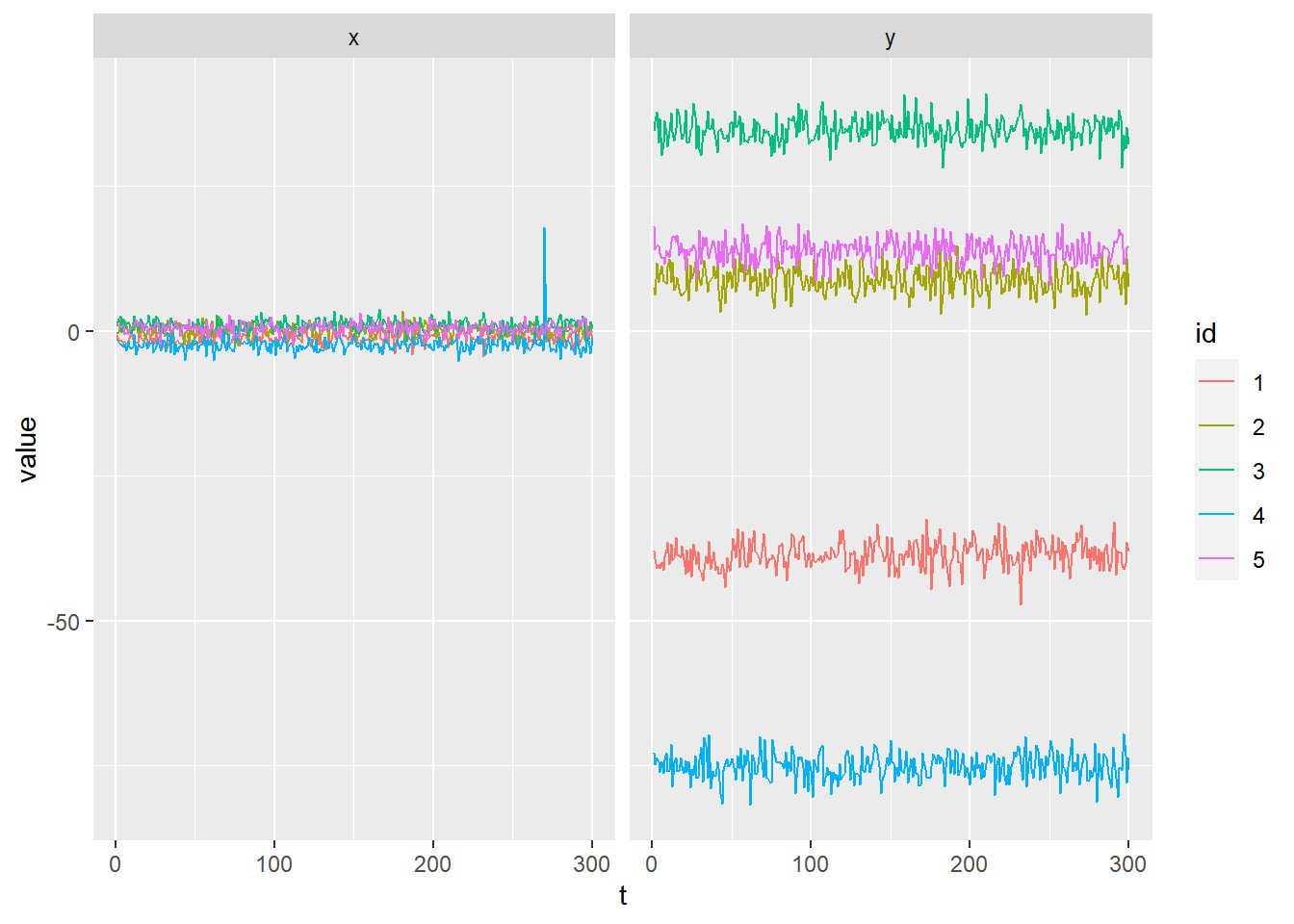B. Specific Outlier in y

B <- fulldata
B %>%
mutate(y = ifelse(id == 2 & t== 80,y+20,y)) -> B

B %>%
select(id,t,x,y) %>%
mutate(id = factor(id)) %>%
pivot_longer(cols = c(-id,-t),names_to="variable",values_to ="value") %>%

ggplot(aes(x=t,y=value,color=id)) +
geom_line() +
facet_wrap(~variable)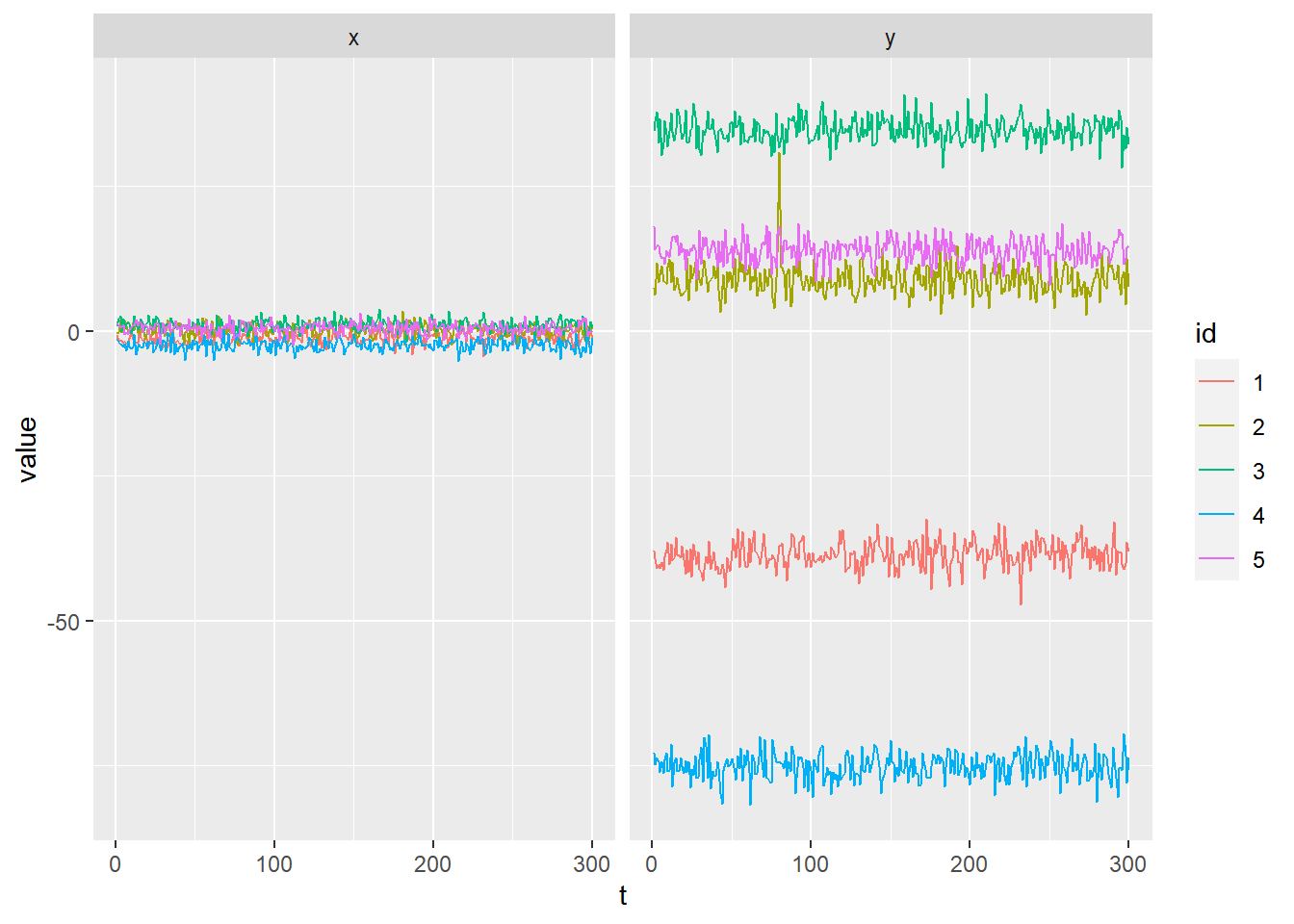C. Common Outlier in x

C <- fulldata
C %>%
mutate(x = ifelse(t==120,x+20,x)) -> C

C %>%
select(id,t,x,y) %>%
mutate(id = factor(id)) %>%
pivot_longer(cols = c(-id,-t),names_to="variable",values_to ="value") %>%

ggplot(aes(x=t,y=value,color=id)) +
geom_line() +
facet_wrap(~variable)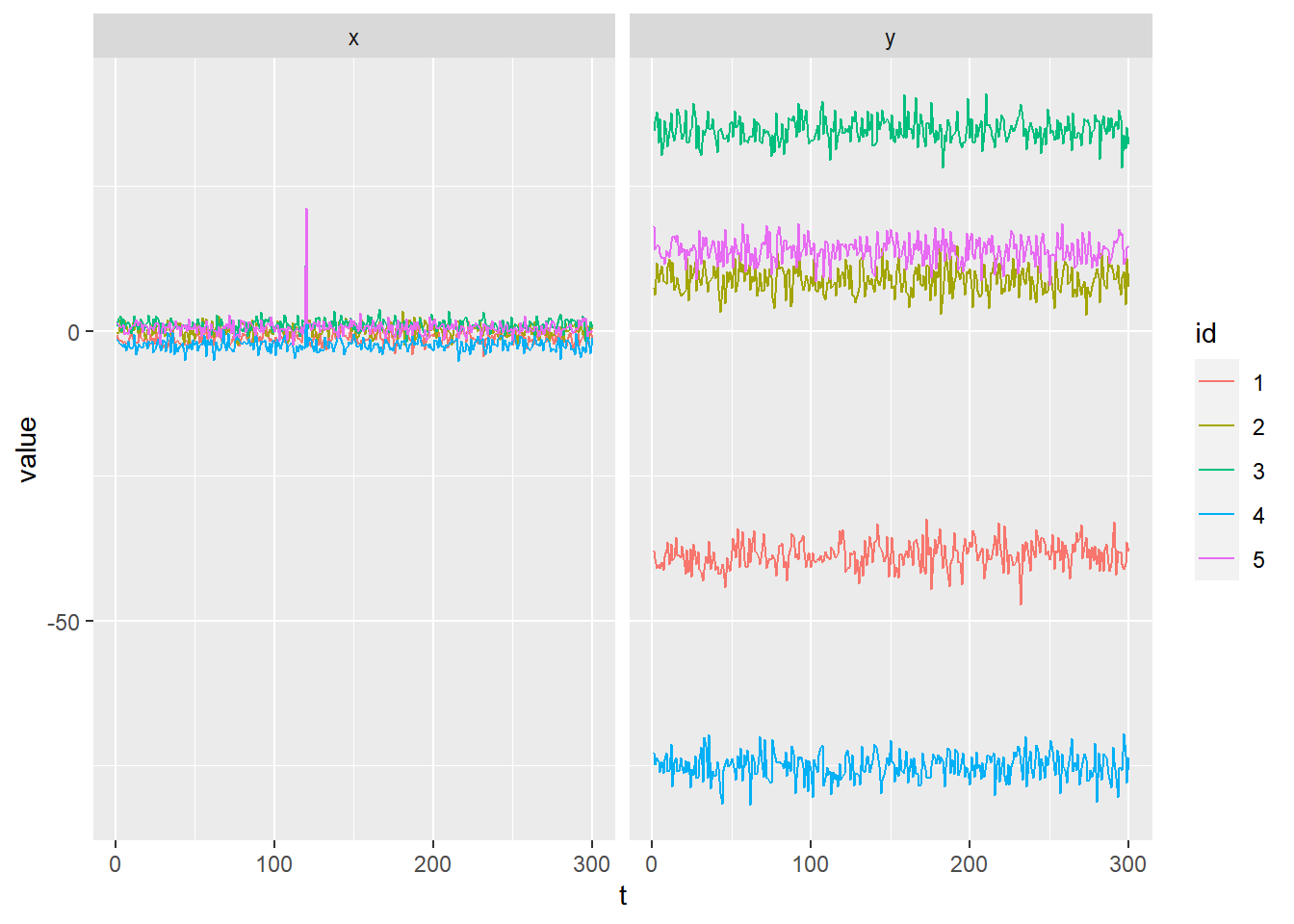D. Common Outlier in y

fulldata %>%
mutate(y = ifelse(t==240,y+20,y)) -> D

D %>%
select(id,t,x,y) %>%
mutate(id = factor(id)) %>%
pivot_longer(cols = c(-id,-t),names_to="variable",values_to ="value") %>%

ggplot(aes(x=t,y=value,color=id)) +
geom_line() +
facet_wrap(~variable)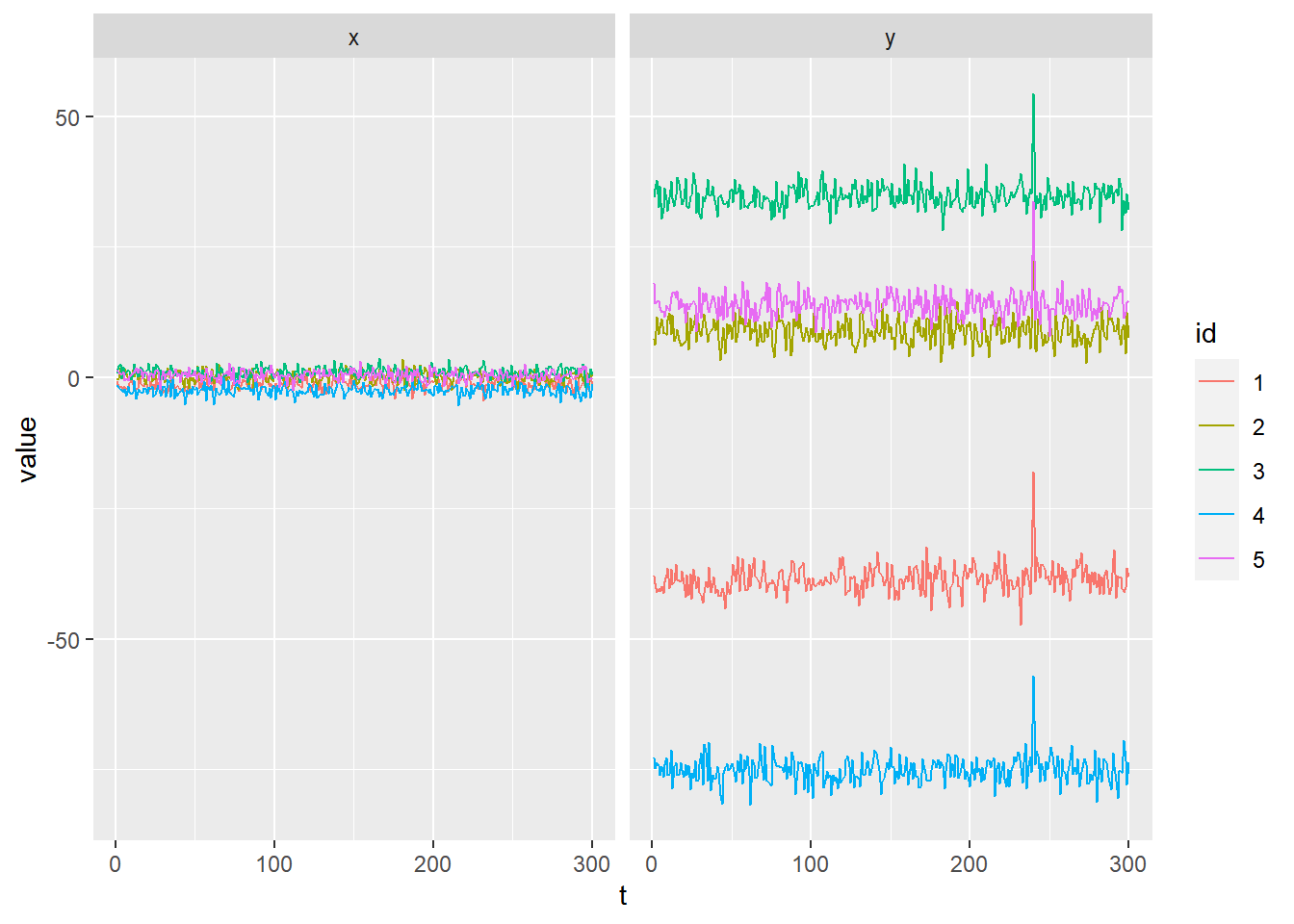# Step Shifts

E. Specific Step-Shift in x

fulldata %>%
mutate(x = ifelse(id == 4 & t> 270,x+20,x)) -> E

E %>%
select(id,t,x,y) %>%
mutate(id = factor(id)) %>%
pivot_longer(cols = c(-id,-t),names_to="variable",values_to ="value") %>%

ggplot(aes(x=t,y=value,color=id)) +
geom_line() +
facet_wrap(~variable)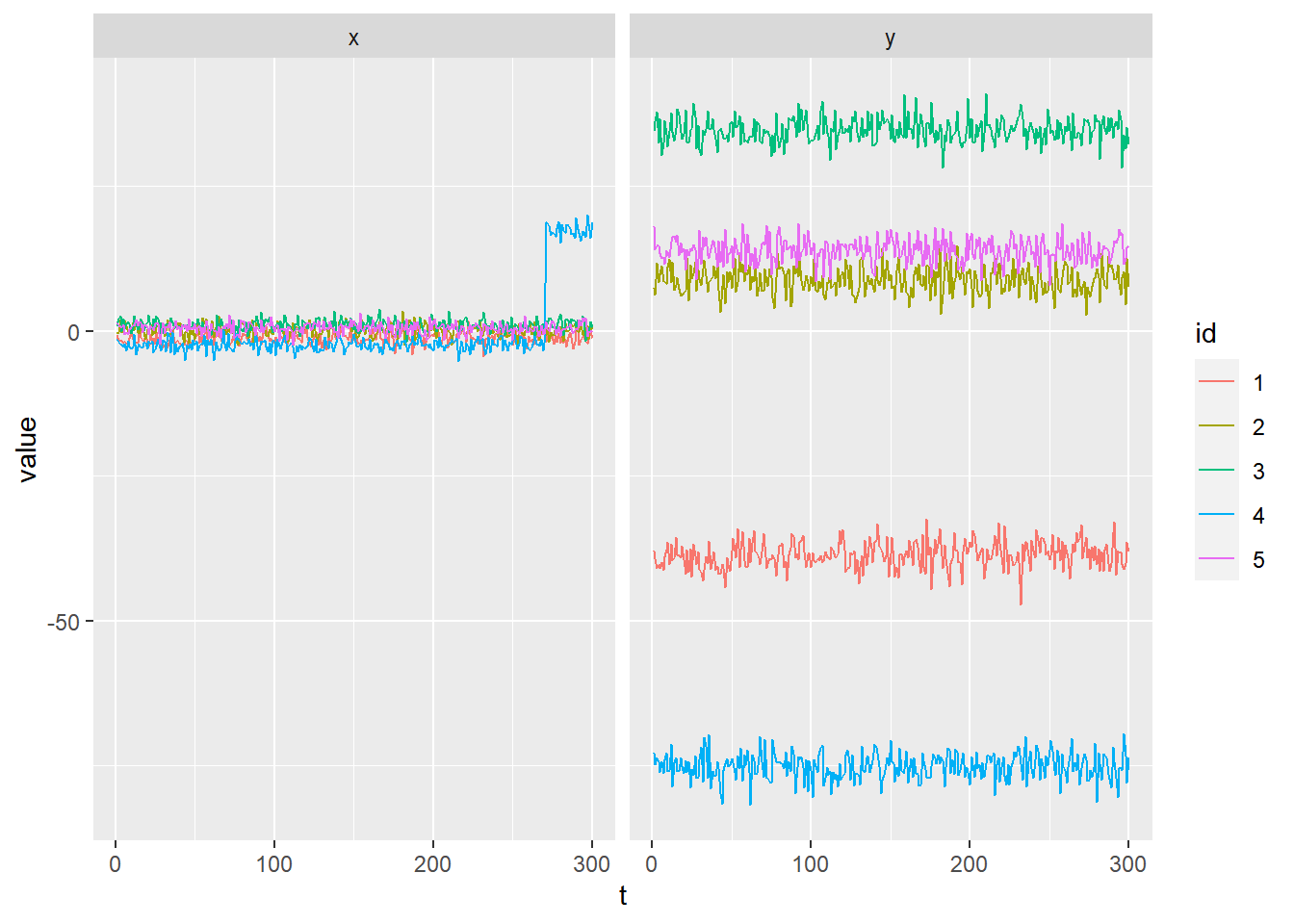G. Specific Step-Shift in y

fulldata %>%
mutate(y = ifelse(id == 2 & t> 80,y+20,y)) -> G

G %>%
select(id,t,x,y) %>%
mutate(id = factor(id)) %>%
pivot_longer(cols = c(-id,-t),names_to="variable",values_to ="value") %>%

ggplot(aes(x=t,y=value,color=id)) +
geom_line() +
facet_wrap(~variable)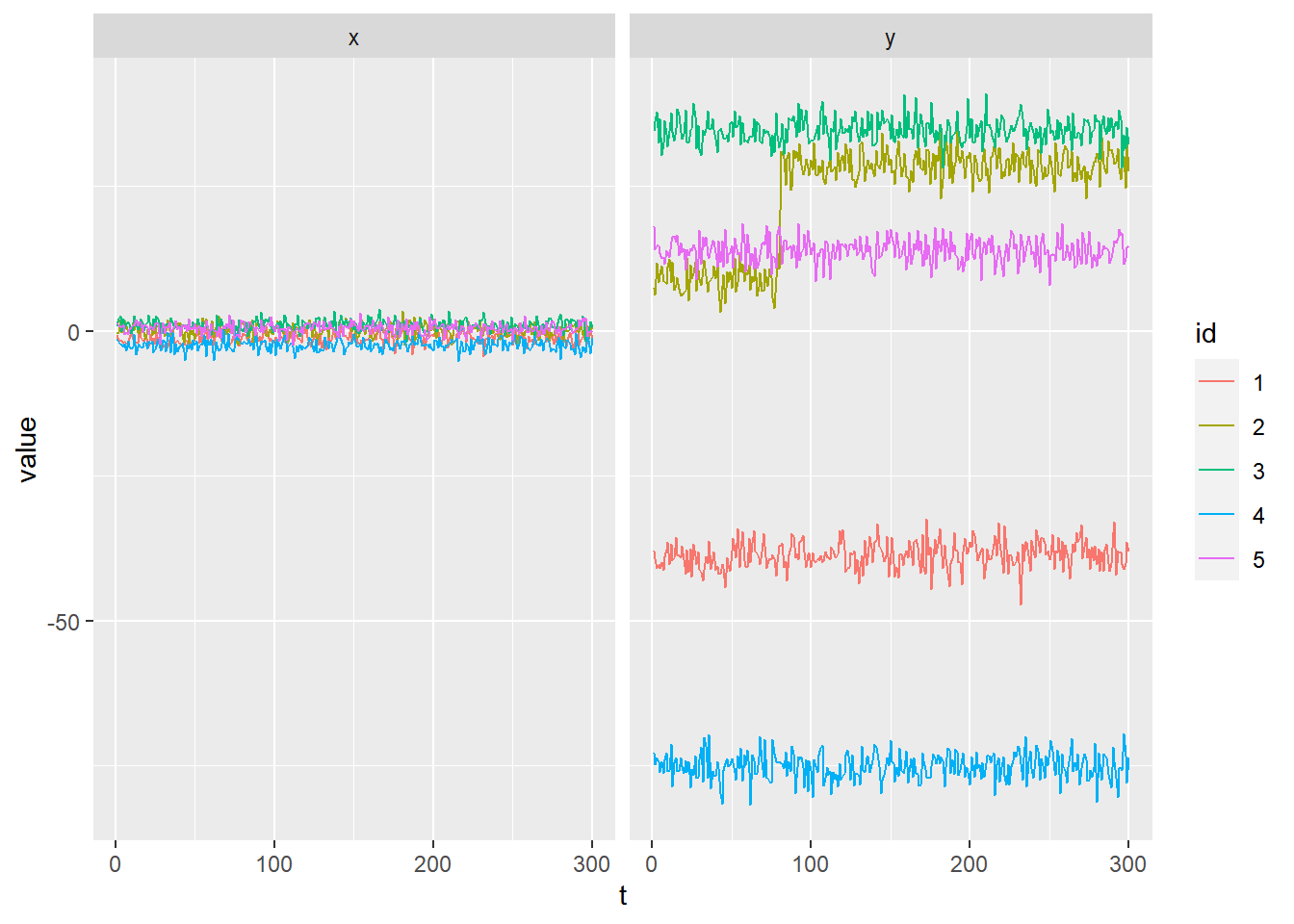## H. Common Outlier in x

fulldata %>%
mutate(x = ifelse(t>120,x+20,x)) -> H

H %>%
select(id,t,x,y) %>%
mutate(id = factor(id)) %>%
pivot_longer(cols = c(-id,-t),names_to="variable",values_to ="value") %>%

ggplot(aes(x=t,y=value,color=id)) +
geom_line() +
facet_wrap(~variable)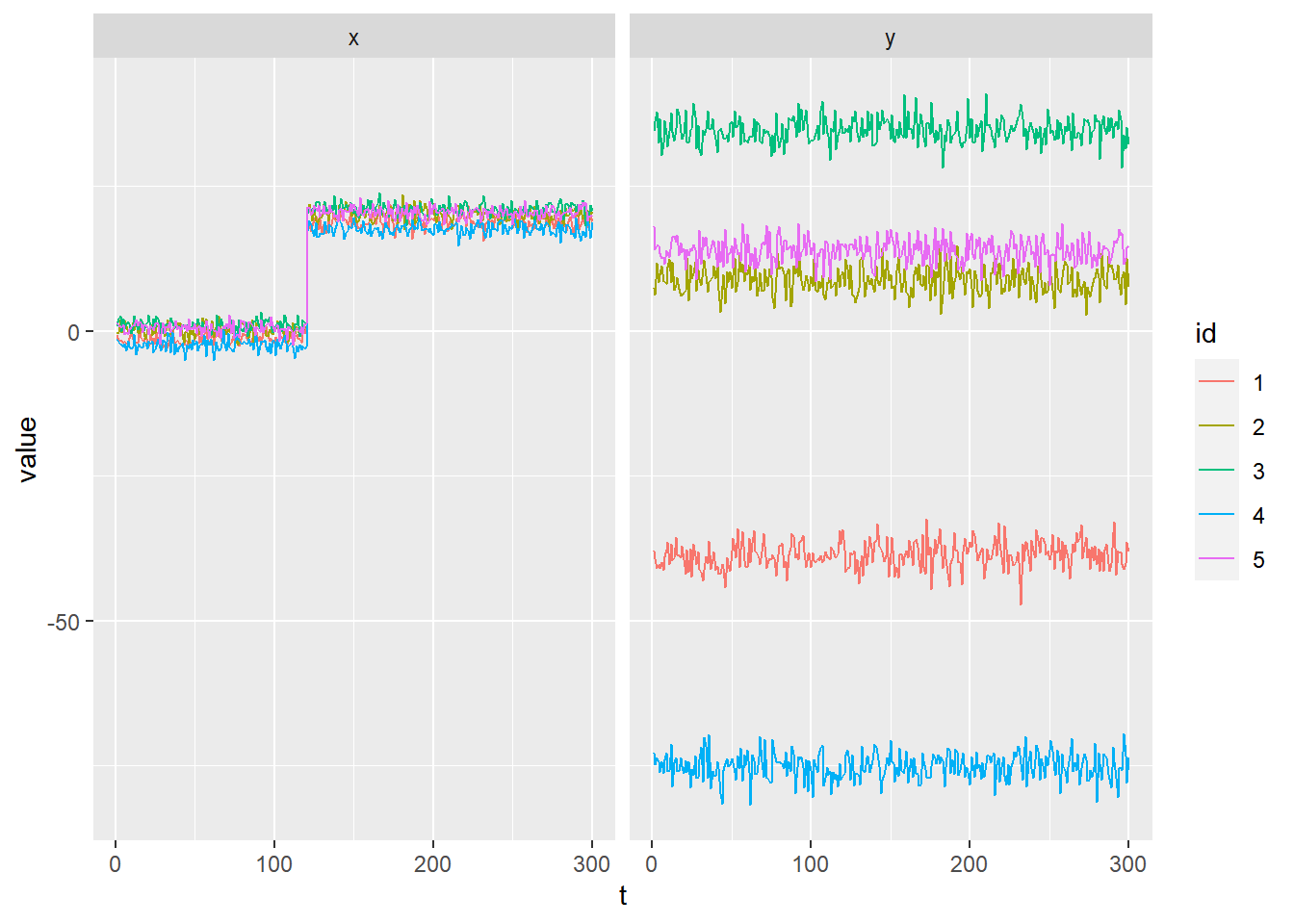I. Common Outlier in y

fulldata %>%
mutate(y = ifelse(t>240,y+20,y)) -> I

I %>%
select(id,t,x,y) %>%
mutate(id = factor(id)) %>%
pivot_longer(cols = c(-id,-t),names_to="variable",values_to ="value") %>%

ggplot(aes(x=t,y=value,color=id)) +
geom_line() +
facet_wrap(~variable)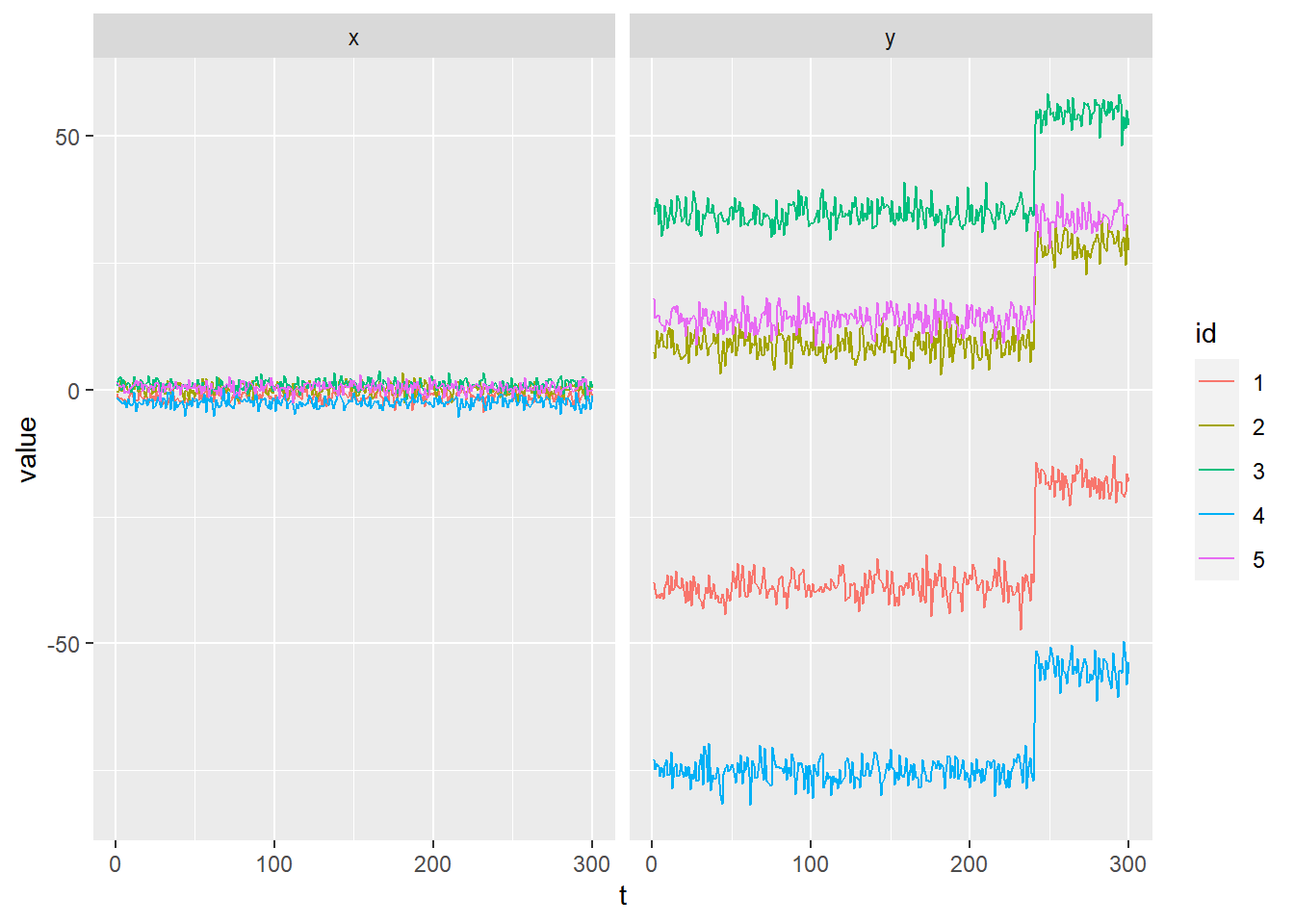Coefficient Shift

shift_factor <- 3

Common Coefficient Shift

fulldata %>%
mutate(y= ifelse(test = t>190,
yes = (true_beta*shift_factor)*fulldata$x + fe_coefficient*fulldata$fe + fulldata\$u,no = y)) %>%
select(id,t,x,y) %>%
mutate(id = factor(id)) %>%
pivot_longer(cols = c(-id,-t),names_to="variable",values_to ="value") %>%

ggplot(aes(x=t,y=value,color=id)) +
geom_line() +
facet_wrap(~variable)